• +91 9971497814
• info@interviewmaterial.com

# Chapter 6 Lines and Angles Ex-6.2 Interview Questions Answers

### Related Subjects

Question 1 : In figure, find the values of x and y and then show that AB || CD.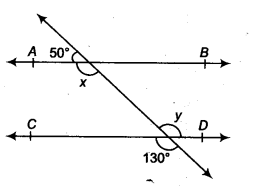In the figure, we have CD and PQ intersect at F.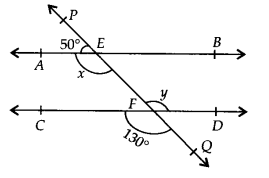∴ y = 130° …(1)
[Vertically opposite angles]
Again, PQ is a straight line and EA stands on it.
∠AEP + ∠AEQ = 180° [Linear pair]
or 50° + x = 180°
⇒ x = 180° – 50° = 130° …(2)
From (1) and (2), x = y
As they are pair of alternate interior angles.
∴ AB || CD

Question 2 : In figure, if AB || CD, CD || EF and y : z = 3 : 7, find x.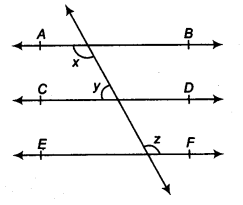AB || CD, and CD || EF [Given]
∴ AB || EF
∴ x = z [Alternate interior angles] ….(1)
Again, AB || CD
⇒ x + y = 180° [Co-interior angles]
⇒ z + y = 180° … (2) [By (1)]
But y : z = 3 : 7
z = 7/3 y = 7/3(180°- z) [By (2)]
⇒ 10z = 7 x 180°
⇒ z = 7 x 180° /10 = 126°
From (1) and (3), we have
x = 126°.

Question 3 : In figure, if AB || CD, EF ⊥ CD and ∠GED = 126°, find ∠AGE, ∠GEF and ∠FGE.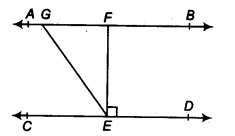AB || CD and GE is a transversal.
∴ ∠AGE = ∠GED [Alternate interior angles]
But ∠GED = 126° [Given]
∴∠AGE = 126°
Also, ∠GEF + ∠FED = ∠GED
or ∠GEF + 90° = 126° [∵ EF ⊥ CD (given)]
x = z [Alternate interior angles]… (1) Again, AB || CD
⇒ x + y = 180° [Co-interior angles]
∠GEF = 126° -90° = 36°
Now, AB || CD and GE is a transversal.
∴ ∠FGE + ∠GED = 180° [Co-interior angles]
or ∠FGE + 126° = 180°
or ∠FGE = 180° – 126° = 54°
Thus, ∠AGE = 126°, ∠GEF=36° and ∠FGE = 54°.

Question 4 : In figure, if PQ || ST, ∠ PQR = 110° and ∠ RST = 130°, find ∠QRS.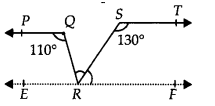Draw a line EF parallel to ST through R.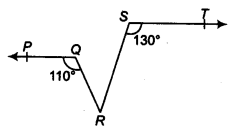Since PQ || ST [Given]
and EF || ST [Construction]
∴ PQ || EF and QR is a transversal
⇒ ∠PQR = ∠QRF [Alternate interior angles] But ∠PQR = 110° [Given]
∴∠QRF = ∠QRS + ∠SRF = 110° …(1)
Again ST || EF and RS is a transversal
∴ ∠RST + ∠SRF = 180° [Co-interior angles] or 130° + ∠SRF = 180°
⇒ ∠SRF = 180° – 130° = 50°
Now, from (1), we have ∠QRS + 50° = 110°
⇒ ∠QRS = 110° – 50° = 60°
Thus, ∠QRS = 60°.

Question 5 : In figure, if AB || CD, ∠APQ = 50° and ∠PRD = 127°, find x and y.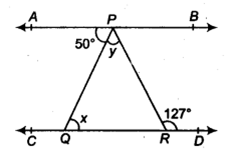We have AB || CD and PQ is a transversal.
∴ ∠APQ = ∠PQR
[Alternate interior angles]
⇒ 50° = x [ ∵ ∠APQ = 50° (given)]
Again, AB || CD and PR is a transversal.
∴ ∠APR = ∠PRD [Alternate interior angles]
⇒ ∠APR = 127° [ ∵ ∠PRD = 127° (given)]
⇒ ∠APQ + ∠QPR = 127°
⇒ 50° + y = 127° [ ∵ ∠APQ = 50° (given)]
⇒ y = 127°- 50° = 77°
Thus, x = 50° and y = 77°.

Question 6 : In figure, PQ and RS are two mirrors placed parallel to each other. An incident ray AB strikes the mirror PQ at B, the reflected ray moves along the path BC and strikes the mirror RS at C and again reflects back along CD. Prove that AB || CD.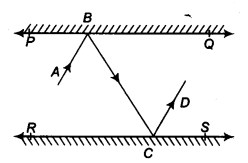Draw ray BL ⊥PQ and CM ⊥ RS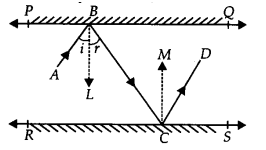∵ PQ || RS ⇒ BL || CM
[∵ BL || PQ and CM || RS]
Now, BL || CM and BC is a transversal.
∴ ∠LBC = ∠MCB …(1) [Alternate interior angles]
Since, angle of incidence = Angle of reflection
∠ABL = ∠LBC and ∠MCB = ∠MCD
⇒ ∠ABL = ∠MCD …(2) [By (1)]
Adding (1) and (2), we get
∠LBC + ∠ABL = ∠MCB + ∠MCD
⇒ ∠ABC = ∠BCD
i. e., a pair of alternate interior angles are equal.
∴ AB || CD.

Todays Deals### Chapter 6 Lines and Angles Ex-6.2 Contributorskrishan

Name:
Email:

# Latest News# 9000 interview questions in different categories